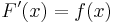# Using Mediawiki In The Classroom

• I find it very easy to develop then deliver lectures with Mediawiki
• Can copy and paste text and images from other Mediawiki websites (e.g. Wikipedia) provided the license allows (generally OK with Wikipedia text, often OK with images, but see license)
• Mediawiki has well tested, powerful features, (It runs Wikipedia),

Including:

• Tex formula, e.g. fundamental theorem of calculus (copied from here):
Let ƒ be a continuous real-valued function defined on a closed interval [a, b]. Let F be the function defined, for all x in [a, b], by$F(x) = \int_a^x f(t)\, dt\,.$
Then, F is continuous on [a, b], differentiable on the open interval (a, b), and$F'(x) = f(x)\,$
for all x in (a, b).